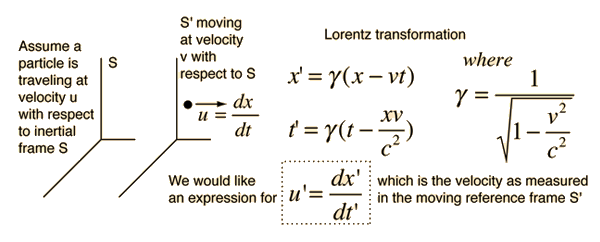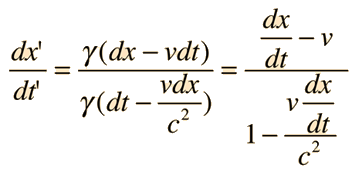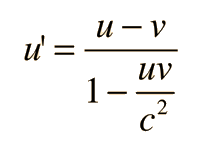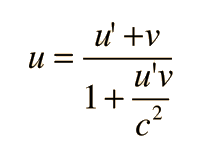# Relativistic Velocity Transformation

No two objects can have a relative velocity greater than c! But what if I observe a spacecraft traveling at 0.8c and it fires a projectile which it observes to be moving at 0.7c with respect to it!? Velocities must transform according to the Lorentz transformation, and that leads to a very non-intuitive result called Einstein velocity addition.Just taking the differentials of these quantities leads to the velocity transformation. Taking the differentials of the Lorentz transformation expressions for x' and t' above givesPutting this in the notation introduced in the illustration above:The reverse transformation is obtained by just solving for u in the above expression. Doing that givesApplying this transformation to the spacecraft traveling at 0.8c which fires a projectile which it observes to be moving at 0.7c with respect to it, we obtain a velocity of 1.5c/1.56 = 0.96c rather than the 1.5c which seems to be the common sense answer.

 Basic application Relativistic relative velocity Velocity of projectile, external observer Velocity of projectile from target
Index

 HyperPhysics***** Relativity R Nave
Go Back